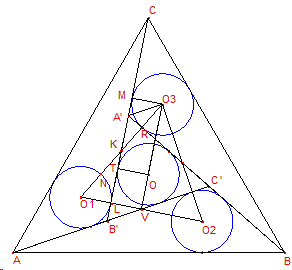# Four Incircles in an Equilateral Triangle, a Sangaku

Elsewhere we described and solved the following sangaku:In an equilateral triangle $ABC$ the lines $AB'C'$, $BC'A'$, $CA'B'$ are drawn making equal angles with $AB$, $BC$, $CA$, respectively, forming the triangle $A'B'C'$, and so that the radius of the incircle of triangle $A'B'C'$ is equal to the radius of the incircle of triangle $AC'B$. Find $A'B'$ in terms of $AB$.

Angela Drei, an Italian math teacher, has supplied (August 4, 2012) an additional proof that also confirms an observation about three pairs of parallel lines made on the original page.

Angela's proof also proceeds in steps.

### Proposition 1

The triangle $O_{1}O_{2}O_{3}$ is equilateral.

Proof: $\Delta ABC'$ is obtained from $ACB'$ by a $120^{\circ}$ counter-clockwise rotation about the center of $ABC$ and similarly $CA'B$ is obtained by $ABC'$, $O_{1}$ is the center of the incircle of triangle $AB'C$, by the previous rotation we obtain the centers $O_{2}$ and $O_{3}$, then the triangle $O_{1}O_{2}O_{3}$ is equilateral.

### Proposition 2

$L$ (point of tangency) $\in O_{1}O_{2}$. (Similarly, $R\in O_{1}O_{3}$, where $R$ is another point of tangency.)

Proof: $OT$ and $O_{3}M$ are perpendicular to $CB'$, $OO_{3}$ and $MT$ are parallel because the distances $OT$ and $O_{3}M$ are equal, so $\angle MKO_{3}=\angle KO_{3}O=30^{\circ}$, $L$ is the point of intersection of $MT$ and $O_{1}O_{2}$, but $O_{3}O$ is perpendicular to $O_{1}O_{2}$ then $ML$ is perpendicular to $O_{1}O_{2}$, showing that $L$ is the point of tangency.

### Proposition 3

The side of $O_{1}O_{2}O_{3}$ is four times $r$, the radius of the incircles.

Proof: in right triangles $O_{1}LK$ and $O_{3}MK$, $\angle MKO_{3}=\angle O_{1}KL$, as vertically opposite, and $O_{3}M=O1L=r$, so that the triangles are congruent and $K$ is the midpoint of $O_{1}O_{3}$, $\angle O_{1}KL=30^{\circ}$ as was already shown, and $\angle KO_{1}L=\angle MO_{3}K=60^{\circ}$. Further, $\angle A'O_{3}M=30^{\circ}$; triangles $A'RK$ and $A'RO_{3}$ are congruent. Indeed, they have $A'R$ as common side, and $A'R$ is perpendicular to $KO_{3}$, making $O_{1}O_{3}=4r$.

Now let $A'B'=l$; $l=A'K+KL+LB'$ and, as a consequence of the previous claims, we deduce $A'K=\frac{2}{\sqrt{3}}KR=\frac{2}{3}\sqrt{3}r$, $LB'=\frac{1}{2}\left(\frac{2}{\sqrt{3}}r\right)=\frac{1}{3}\sqrt{3}r$, and $KL=\frac{\sqrt{3}}{2}2r=\sqrt{3}r$, so that $l=2\sqrt{3}r$.

We consider the areas of the four triangles that form $\triangle ABC$; the sum of their areas equals that of $\triangle ABC$, but each can be calculated separately. The area of $\triangle AB'C$ is $xr+\frac{\sqrt{3}}{3}r^{2}$, where $x$ is the side of $\triangle ABC$. The area of $\triangle A'B'C'$ is $\frac{3}{2}lr$ and also $l^{2}\frac{\sqrt{3}}{4}$, making $r=l\frac{\sqrt{3}}{6}$. The area of $\triangle ABC=x^{2}\frac{\sqrt{3}}{4}$. Summing up leads to the equation

$3xr + \sqrt{3}r^{2} + \frac{3}{2}lr=\frac{x^{2}\sqrt{3}}{4}.$

Replacing $r=l\frac{\sqrt3}}{6} we obtain \(3xl\frac{\sqrt{3}}{6} + l^{2}\frac{\sqrt{3}}{12} + \frac{\sqrt{3}}{4}l^{}=\frac{x^{2}\sqrt{3}}{4}.$

Or, after simplification $6xl+4l^{2}-3x^{2}=0$, with the only positive root $l=x\frac{\sqrt{21}-3}{4}$.

(There is another proof by George Zettler and a third one by J. John Samuel.)